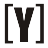# Amps (A) to kilovolt-amps (kVA) Calculator

To calculate Amps to kilovolt-amps, select phase (single or three phase) first, insert value of Amps and volts then press Calculate Button to get the result of kilovolt-amps (kVA). Swap to kVA to Amps calculator.

 Select phase #: Single phase Three phase Enter amps: A Enter volts: V Result in kilovolt-amps: kVA

## How to Calculate Amps (A) to kilovolt-amps (kVA)

Single phase amps to kVA Formula
`S (kVA) = I (A) × V (V) / 1000`

Power S in kilovolt-amps equal to current I in amps, multiply the voltage V in volts, divided by 1000.

Three phase amps to kVA Formula
Line to line voltage

`S (kVA) = √3 × I (A) × VL-L (V) / 1000`

Power S in kilovolt-amps equal to phase current I in amps, multiply the line to line RMS voltage VL-L in volts, divided by 1000.

Line to neutral voltage

`S (kVA) = 3 × I (A) × VL-N (V) / 1000`

Power S in kilovolt-amps equal to phase current I in amps, multiply the line to neutral RMS voltage VL-N in volts, divided by 1000.YoosFuhl.com Would you like to receive notifications on latest updates? No Yes